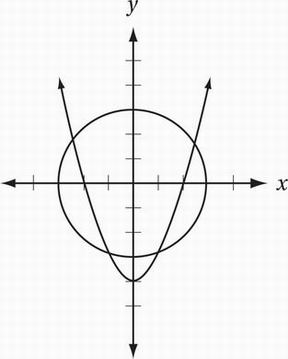# SAT Math Multiple Choice Question 602: Answer and Explanation

### Test Information

Question: 602

2.x2 + y2 = 9

y = x2 - 4

A system of two equations and their graphs in the xy-plane are shown above. How many solutions does the system have?

• A. One
• B. Two
• C. Three
• D. Four